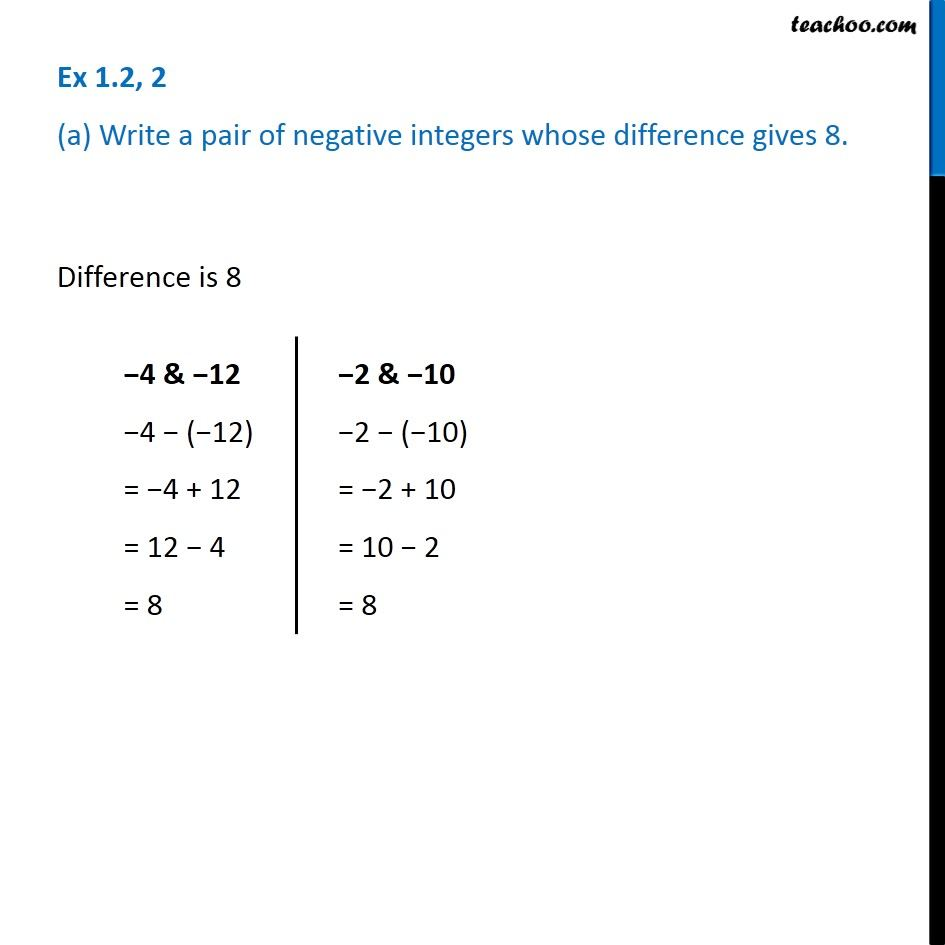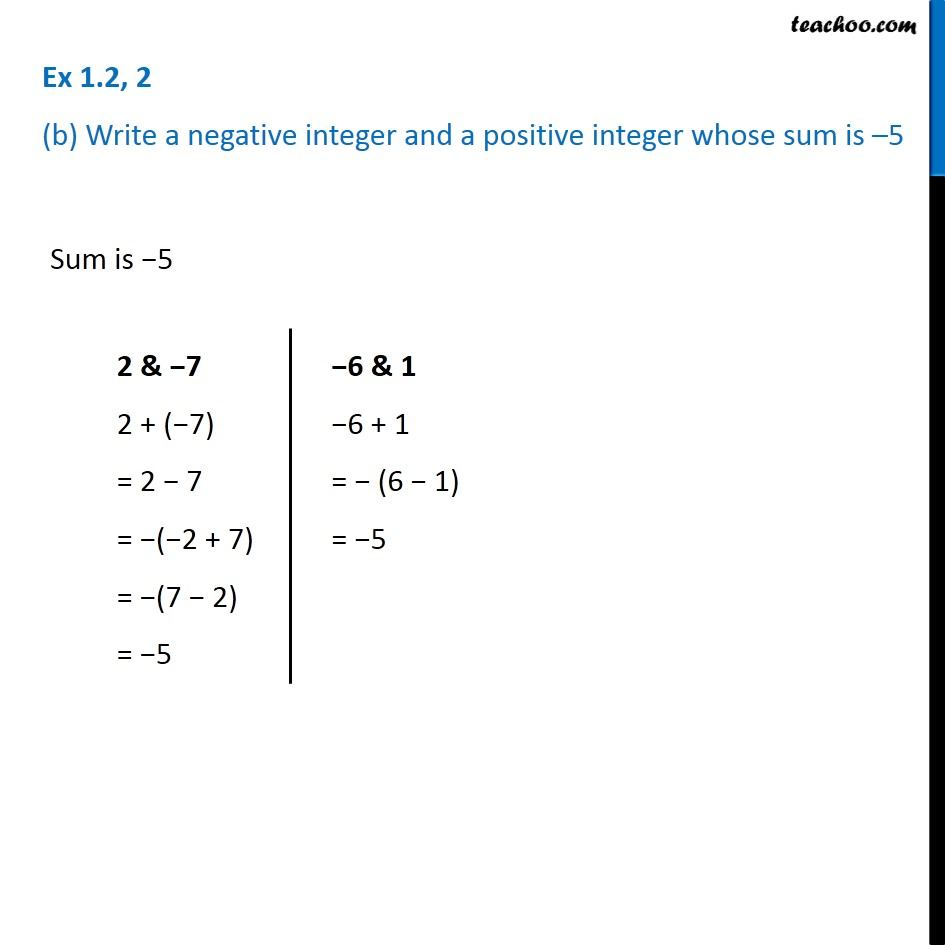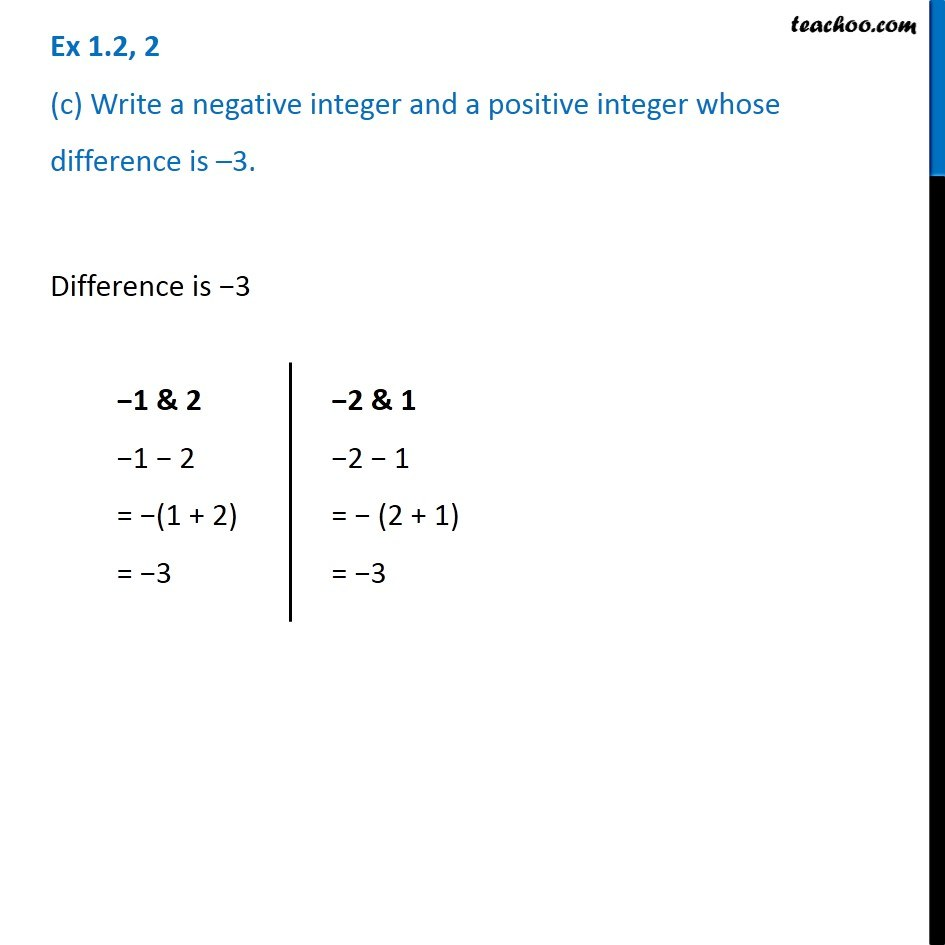Subscribe to our Youtube Channel - https://you.tube/teachoo

1. Chapter 1 Class 7 Integers
2. Serial order wise
3. Ex 1.2

Transcript

Ex 1.2, 2 (a) Write a pair of negative integers whose difference gives 8. Difference is 8 4 & 12 4 ( 12) = 4 + 12 = 12 4 = 8 2 & 10 2 ( 10) = 2 + 10 = 10 2 = 8 Ex 1.2, 2 (b) Write a negative integer and a positive integer whose sum is 5 Sum is 5 2 & 7 2 + ( 7) = 2 7 = ( 2 + 7) = (7 2) = 5 6 & 1 6 + 1 = (6 1) = 5 Ex 1.2, 2 (c) Write a negative integer and a positive integer whose difference is 3. Difference is 3 1 & 2 1 2 = (1 + 2) = 3 2 & 1 2 1 = (2 + 1) = 3

Ex 1.2

Chapter 1 Class 7 Integers
Serial order wise